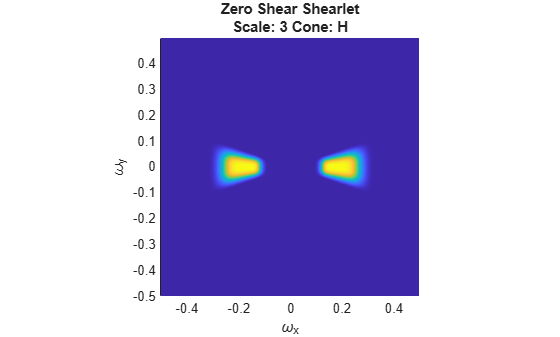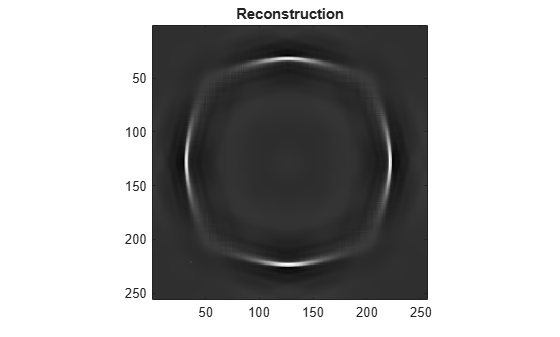Main Content

# sheart2

Shearlet transform

## Syntax

coefs = sheart2(sls,im)

## Description

example

coefs = sheart2(sls,im) returns the shearlet transform or shearlet analysis of the real-valued 2-D image im for the shearlet system sls. If the shearlet system is real-valued with periodic boundary conditions, then coefs is real-valued. Otherwise, coefs is complex-valued. The size and class (data type) of im must match the ImageSize and Precision values, respectively, of sls.

## Examples

collapse all

This example shows how to take the shearlet transform of an image and reconstruct the image using only coefficients corresponding to zero shearing.

Load and display an image of a circle.

load circleGS imagesc(circleGS) colormap gray axis equal axis tightCreate a shearlet system that can be used with the image. Obtain the shearlet filters defined by the system, as well as their geometric interpretations.

[numRows,numCols] = size(circleGS); sls = shearletSystem('ImageSize',[numRows numCols],'FilterBoundary','truncated'); [psi,scale,shear,cone] = filterbank(sls);

Obtain the shearlet transform of the image.

cfs = sheart2(sls,circleGS);

Find the indices of the shearlet filters that correspond to zero shearing. Keep in mind that the lowpass filter also corresponds to zero shearing.

ind = find((shear==0).*(scale~=-1))'
ind = 1×10 3 6 10 15 20 25 31 38 46 55 

Plot one of the shearlets in the frequency plane. Because the shearlet corresponds to zero shearing, confirm the frequency response is concentrated along either the horizontal or vertical axis.

sh = 31; omegax = -1/2:1/numCols:1/2-1/numCols; omegay = omegax; figure surf(omegax,flip(omegay),psi(:,:,sh),'EdgeColor','none') view(0,90) xlabel('\omega_x') ylabel('\omega_y') axis equal axis tight title(['Zero Shear Shearlet: Scale = ',num2str(scale(sh)),', Cone - ',cone{sh}])Create an array that only contains the shearlet coefficients that correspond to the zero shearing filters.

cfsx = zeros(size(cfs)); for k=1:length(ind) cfsx(:,:,ind(k)) = cfs(:,:,ind(k)); end

Reconstruct the image using the new coefficients array. Because the only nonzero shearlet coefficients are those that correspond to zero shearing, the horizontal and vertical portions of the circle are emphasized in the reconstruction.

rec = isheart2(sls,cfsx); imagesc(rec) axis equal axis tight colormap gray title('Reconstruction')## Input Arguments

collapse all

Shearlet system, specified as a shearletSystem object.

Input image, specified a real-valued matrix. The size and data type of im must match the ImageSize and Precision values, respectively, of sls.

Data Types: single | double

## Output Arguments

collapse all

Shearlet coefficients, returned as a 3-D array. The size of coefs is M-by-N-by-K, where M and N are the row and column dimensions of the input image, respectively. The size of the third dimension, K, equals the number of shearlets in sls, including the lowpass filter, K = numshears(sls) + 1.

For example, if cfs = sheart2(sls,im) and psi = filterbank(sls), then the shearlet corresponding to cfs(:,:,k) is psi(:,:,k). The data type of coefs matches the Precision value of the shearlet system.

Data Types: single | double

## See Also

### Topics

Introduced in R2019b

## SupportGet trial now